# Principal homogeneous space

A principal-object in the category of algebraic varieties or schemes. Ifis a scheme andis a group scheme over, then a principal-object in the category of schemes overis said to be a principal homogeneous space. Ifis the spectrum of a field(cf. Spectrum of a ring) andis an algebraic-group (cf. Algebraic group), then a principal homogeneous space overis an algebraic-varietyacted upon (from the left) bysuch that ifis replaced by its separable algebraic closure, then each point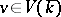defines an isomorphic mapping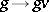of the varietiesand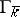. A principal homogeneous spaceis trivial if and only if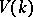is non-empty. The set of classes of isomorphic principal homogeneous spaces over a smooth algebraic groupcan be identified with the set of Galois cohomology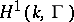. In the general case the set of classes of principal homogeneous spaces over an-group schemecoincides with the set of one-dimensional non-Abelian cohomology. Here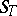is some Grothendieck topology on the scheme.
Principal homogeneous spaces have been computed in a number of cases. Ifis a finite field, then each principal homogeneous space over a connected algebraic-group is trivial (Lang's theorem). This theorem also holds ifis a-adic number field andis a simply-connected semi-simple group (Kneser's theorem). If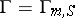is a multiplicative-group scheme, then the set of classes of principal homogeneous spaces overbecomes identical with the Picard group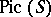of. In particular, ifis the spectrum of a field, this group is trivial. If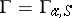is an additive-group scheme, then the set of classes of principal homogeneous spaces overbecomes identical with the one-dimensional cohomology group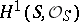of the structure sheaf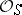of. In particular, this set is trivial ifis an affine scheme. Ifis a global field (i.e. an algebraic number field or a field of algebraic functions in one variable), then the study of the set of classes of principal homogeneous spaces over an algebraic-groupis based on the study of the Tate–Shafarevich set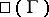, which consists of the principal homogeneous spaces overwith rational points in all completionswith respect to the valuations of. Ifis an Abelian group over the field, then the set of classes of principal homogeneous spaces overforms a group (cf. Weil–Châtelet group).